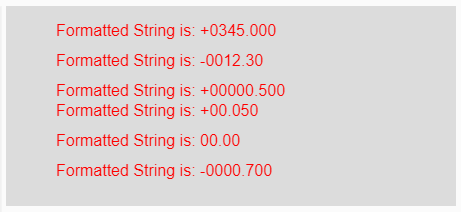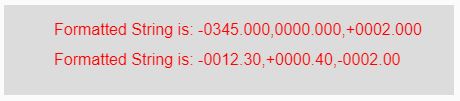# p5.js | nfp() Function

The nfp() function in p5.js is used to format the input numbers (integers or floats) into strings as well as it gives positive (+) and negative (-) sign according to the sign of input numbers.

Syntax:

`nfp(Num, Left, Right)`

Parameters: This function accepts three parameters as mentioned above and described below:

• Num: This is the input positive number or an array of numbers which are to be formatted.
• Left: This is the positive number which says the number of digits should be on the left side of the decimal point.
• Right: This is the positive number which says the number of digits should be on the right side of the decimal point.

Return Value: It returns the formatted string.

Below programs illustrate the nfp() function in p5.js:

Example 1: This example uses nfp() function to format the input numbers.

 `function` `setup() {  ` `  `  `    ``// Creating Canvas size ` `    ``createCanvas(450, 200);  ` `}  ` ` `  `function` `draw() {  ` `      `  `    ``// Set the background color  ` `    ``background(220);  ` `    `  `    ``// Initializing the Numbers ` `    ``let num1 = 345;  ` `    ``let num2 = -12.3;  ` `    ``let num3 = .5;  ` `    ``let num4 = .05;  ` `    ``let num5 = 0;  ` `    ``let num6 = -0.7;  ` `     `  `    ``// Calling to nfp() function. ` `    ``let A = nfp(num1, 4, 3); ` `    ``let B = nfp(num2, 4, 2); ` `    ``let C = nfp(num3, 5, 3); ` `    ``let D = nfp(num4, 2, 3); ` `    ``let E = nfp(num5, 2, 2); ` `    ``let F = nfp(num6, 4, 3); ` `     `  `    ``// Set the size of text  ` `    ``textSize(16);  ` `      `  `    ``// Set the text color  ` `    ``fill(color(``'red'``));  ` `    `  `    ``// Getting formatted String ` `    ``text(``"Formatted String is: "` `+ A, 50, 30); ` `    ``text(``"Formatted String is: "` `+ B, 50, 60); ` `    ``text(``"Formatted String is: "` `+ C, 50, 90); ` `    ``text(``"Formatted String is: "` `+ D, 50, 110); ` `    ``text(``"Formatted String is: "` `+ E, 50, 140); ` `    ``text(``"Formatted String is: "` `+ F, 50, 170); ` `}  `

Output:Example 2: This example uses nfp() function to format the input numbers.

 `function` `setup() {  ` `  `  `    ``// Creating Canvas size ` `    ``createCanvas(450, 90);  ` `}  ` ` `  `function` `draw() {  ` `      `  `    ``// Set the background color  ` `    ``background(220);  ` `    `  `    ``// Initializing the array of numbers ` `    ``let num1 = [-345, 0, 2];  ` `    ``let num2 = [-12.3, .4, -2.0];  ` `     `  `    ``// Calling to nfp() function. ` `    ``let A = nfp(num1, 4, 3); ` `    ``let B = nfp(num2, 4, 2); ` `     `  `    ``// Set the size of text  ` `    ``textSize(16);  ` `      `  `    ``// Set the text color  ` `    ``fill(color(``'red'``));  ` `    `  `    ``// Getting formatted String ` `    ``text(``"Formatted String is: "` `+ A, 50, 30); ` `    ``text(``"Formatted String is: "` `+ B, 50, 60); ` `}  `

Output:Reference: https://p5js.org/reference/#/p5/nfpMy Personal Notes arrow_drop_upCheck out this Author's contributed articles.

If you like GeeksforGeeks and would like to contribute, you can also write an article using contribute.geeksforgeeks.org or mail your article to contribute@geeksforgeeks.org. See your article appearing on the GeeksforGeeks main page and help other Geeks.

Please Improve this article if you find anything incorrect by clicking on the "Improve Article" button below.

Article Tags :

Be the First to upvote.

Please write to us at contribute@geeksforgeeks.org to report any issue with the above content.